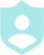# System of Equations Math Jeopardy###### Example QuestionsSolve each system by elimination.Solve each system by elimination.
##### Description :
Solve systems of linear equations using the elimination, substitution, or graphing method.##### Difficulty :GRID 4 Categories 16 QuestionsDownloadPrintShareAdd
Questions
Number of categoriesQuestions per categoryCategory 1
Elimination MethodCategory 2
Substitution MethodCategory 3
GraphingCategory 4
Word ProblemsLayout Preview
 Question Q. Solve each system by elimination. A. (-3, 5) Question Q. Solve each system by substitution. A. (4,8) Question Q. Solve each system by graphing. A. (-1, -1) Question Q. The sum of the digits of a certain two-digit number is 11. When you reverse its digits you decrease the number by 63. What is the number? A. 92 Question Q. Solve each system by elimination. A. (2, 3) Question Q. Solve each system by substitution. A. (-3, -2) Question Q. Solve each system by graphing. A. (-1, 1) Question Q. Heather and Lisa are selling cheesecakes for a school fundraiser. Customers can buy New York style cheesecakes and strawberry cheesecakes. Heather sold 6 New York style cheesecakes and 13 strawberry cheesecakes for a total of \$204. Lisa sold 7 New York style cheesecakes and 1 strawberry cheesecake for a total of \$68. Find the cost each of one New York style cheesecake and one strawberry cheesecake. A. New York style cheesecake: \$8, strawberry cheesecake: \$12 Question Q. Solve each system by elimination. A. (2, -1) Question Q. Solve each system by substitution. A. (-2, 3) Question Q. Solve each system by graphing. A. (2, 4) Question Q. The sum of the digits of a certain two-digit number is 13. When you reverse its digits you decrease the number by 45. Find the number. A. 94 Question Q. Solve each system by elimination. A. (3, -2) Question Q. Solve each system by substitution. A. (-2, 0) Question Q. Solve each system by graphing. A. (-3, 3) Question Q. Jennifer and Castel each improved their yards by planting grass sod and ivy. They bought their supplies from the same store. Jennifer spent \$21 on 1 ft² of grass sod and 3 pots of ivy. Castel spent \$78 on 6 ft² of grass sod and 6 pots of ivy. What is the cost of one ft² of grass sod and the cost of one pot of ivy? A. ft² of grass sod: \$9, pot of ivy: \$4
Colors :
Font color
Button color
Background color
Logo:Background Image:Theme Song: